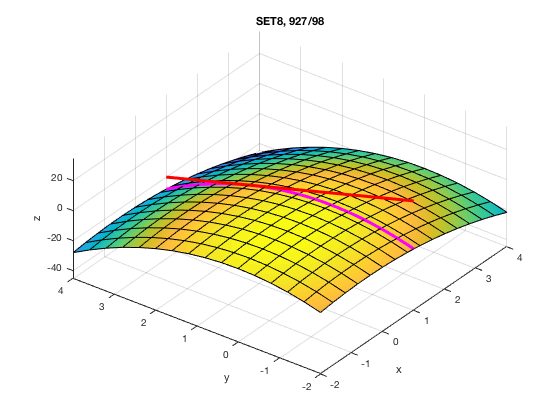# MATH 251: Calculus 3, SET8

## 14: Partial Derivatives

### 14.3: Partial Derivatives

These problems are done with the CAS. See Hand Solutions for details.

#### 1. [924/10]

We estimate the partial derivatives from the contour plot using difference quotients.
f_x_2_1 = (14-10) / (3-2)
f_x_2_1 = 4
f_y_2_1 = (8-10) / (2-1)
f_y_2_1 = -2

#### 2. [924/16]

As well as ordinary derivatives (from Calculus 1), the diff command computes partial derivatives as well.
syms x y
f = x^2*y - 3*y^4
f =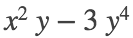fx = diff(f,x), fy = diff(f,y)
fx =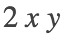fy =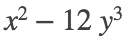#### 3. [924/30]

Recall Part 2 of the Fundamental Theorem of Calculus and Properties of Integrals.
syms alpha beta t
F(alpha,beta) = int(sqrt(t^3 + 1), t, alpha, beta);
F_alpha = simplify(diff(F,alpha)), F_beta = simplify(diff(F,beta))
F_alpha(alpha, beta) =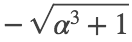F_beta(alpha, beta) =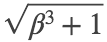#### 4. [924/40]

Here we do an experiment with a specific number of variables,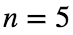.
This may help you to see the general pattern, which is obtained via the Chain Rule.
In general,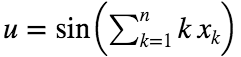whence.
X = sym('x', [1 5])
X =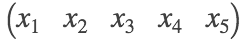u = sin(X(1) + 2*X(2) + 3*X(3) + 4*X(4) + 5*X(5)) % sample with n = 5
u =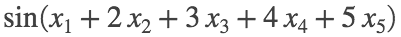M = [];
for j = 1:5
M = [M; [j diff(f, X(j))]];
end
M % column 1: j; column 2: partial derivative of u w.r.t. the jth variable
M =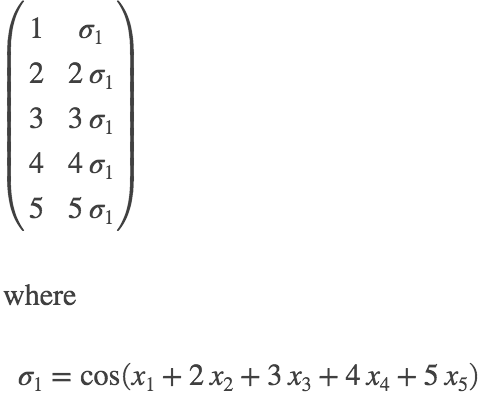#### 5. [925/44]

syms x y z
f(x,y,z) = x^(y*z), fz = diff(f,z), e = exp(sym(1))
f(x, y, z) =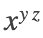fz(x, y, z) =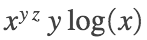e = e
fz_at_P = fz(e,1,0)
fz_at_P = 1

#### 6. [925/50]

Here we look ahead to the end of Section 14.5: The Chain Rule. There we find a much quicker way to do implicit differentiation. See page 943 of textbook.
( It sure beats the Calc 1 way of doing things since its a one-step formula! )
syms x y z
F = y*z + x*log(y) - z^2 % Let F = LHS - RHS of the equation given, understood to be set to zero.
F =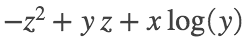zx = -diff(F,x) / diff(F,z)
zx =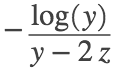zy = -diff(F,y) / diff(F,z)
zy =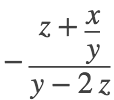#### 7. [925/66]

By hand, you would compute a first, second, and third partial derivative in succession.
That's what the diff command is doing internally and just displaying the final result.
syms r s t
g = exp(r) * sin(s*t), g_rst = simplify( diff(g, r,s,t) )
g =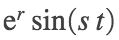g_rst =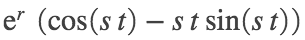#### 8. [925/76]

Let's check all six functions at once! We see that 1st and 3rd functions are not solutions of Laplace's equation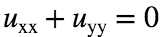; the others are solutions.
syms x y
u = [x^2+y^2 x^2-y^2 x^3+3*x*y^2 1/2*log(x^2+y^2) sin(x)*cosh(y)+cos(x)*sinh(y) exp(-x)*cos(y)-exp(-y)*cos(x)].'
u =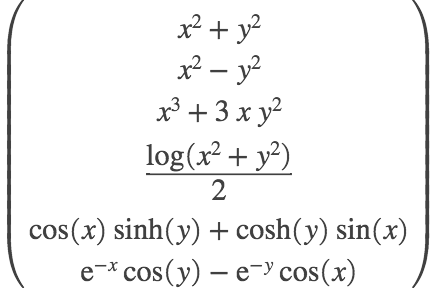L = simplify( diff(u,x,2) + diff(u,y,2) )
L =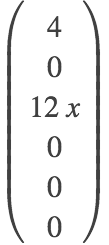#### 9. [926/82]

The requisite partial derivatives are evaluated to determine rates of change of temperature in the specified directions.
syms x y
T(x,y) = 60 / (1+x^2+y^2)
T(x, y) =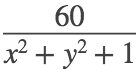Tx = diff(T,x), Ty = diff(T,y)
Tx(x, y) =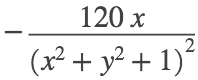Ty(x, y) =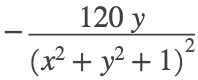Tx_P = Tx(2,1), Ty_P = Ty(2,1)
Tx_P =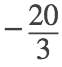Ty_P =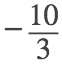#### 10. [927/98]

Parameterize the curve of intersection of the paraboloid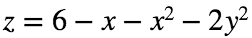and the plane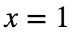as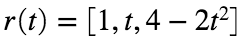. The point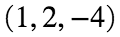corresponds to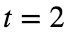. Now proceed in the usual manner.
syms t u x y
r(t) = [1 t 4-2*t^2], Dr = diff(r,t), s1 = sym(1)
r(t) =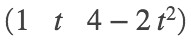Dr(t) =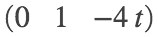s1 = 1
P = r(2), v = Dr(2)
P =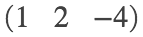v =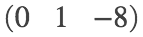L(u) = P + u*v
L(u) =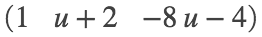z = 6 - x - x^2 - 2*y^2
z =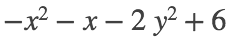%
figure
fsurf(z, [-2 4 -2 4], 'MeshDensity', 16); hold on
fplot3(s1, t, 4-2*t^2, [-2 4], 'm', 'LineWidth', 3)
fplot3(s1, u+2, -8*u-4, [-5 3], 'r', 'LineWidth', 3)
axis([-2 4 -2 4 -45 35])
view(-53,53)
xlabel('x'); ylabel('y'); zlabel('z')
title('SET8, 927/98')